### Notation and Estimation

Let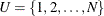be the set of indices and letbe the set of values for a finite population of size N. The survival time of each member of the finite population is assumed to follow its own hazard function,, expressed as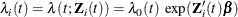where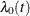is an arbitrary and unspecified baseline hazard function,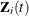is the vector of explanatory variables for the ith unit at time t, andis the vector of unknown regression parameters that are associated with the explanatory variables. The vectoris assumed to be the same for all individuals.

The partial likelihood function introduced by Cox (1972, 1975) eliminates the unknown baseline hazardand accounts for censored survival times. If the entire population is observed, then this partial likelihood can be used to estimate. Letbe the desired estimator. Assuming a working model with uncorrelated responses,is obtained by maximizing the partial log likelihood,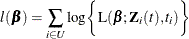with respect to, where Lis Cox’s partial likelihood function.

Assume that probability sample A is selected from the finite population U and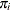is the selection probability for unit i. Further assume that covariatesand survival time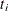are available for every unit in the sample A. An estimator of the finite population log likelihood is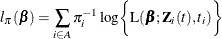See Partial Likelihood Function for the Cox Model for more details.

A sample-based estimator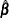for the finite population quantitycan be obtained by maximizing the partial pseudo-log-likelihoodwith respect to. The design-based variance foris obtained by assuming the set of finite population valuesas fixed. For more information about maximum pseudo-likelihood estimators and other inferential approaches for survey data, see Kish and Frankel (1974); Godambe and Thompson (1986); Pfeffermann (1993), Korn and Graubard (1999, chapter 3), Chambers and Skinner (2003, chapter 2), and Fuller (2009, section 6.5). Maximum pseudo-likelihood estimators and their properties for Cox’s proportional hazards model for survey data are discussed in Binder (1990, 1992); Lin and Wei (1989); Lin (2000); Boudreau and Lawless (2006).

Without loss of generality, the rest of this section uses indices for stratified clustered designs. For a stratified clustered sample design, observations are represented by a matrix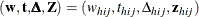where

•denotes the vector of sampling weights

•denotes the event time variable

•denotes the event indicator

•denotes the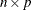matrix of auxiliary information

•is the stratum index

•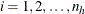is the cluster index within stratum h

•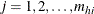is the unit index within cluster i of stratum h

• p is the total number of parameters

•is the total number of observations in the sample

•, whereis an indicator function

•, whereis an indicator function

Let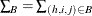denote the summation over the set of indices such that the observation unit j in PSU i and stratum h belongs to the index set B. Typically, B is the set of all population indices that are in the sample, the risk set, or the set of all units with a failure.

The first-stage sampling rate (fraction of PSUs selected for the sample) is denoted by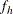. The first-stage sampling rate is used in Taylor series variance estimation. You can specify the stratum sampling rates with the RATE= option. Or if you specify population totals with the TOTAL= option, PROC SURVEYFREQ computesas the ratio of stratum sample size to the stratum total, in terms of PSUs. See the section Population Totals and Sampling Rates for details. If you do not specify the RATE= option or the TOTAL= option, then the procedure assumes that the stratum sampling ratesare negligible and does not use a finite population correction when computing variances.